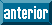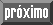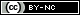## Articulo

•Citado por SciELO
•Accesos

•Similares en SciELO

## versión impresa ISSN 0187-6236

#### Resumen

SKIBA, Yuri N.. Role of forcing in large-time behavior of vorticity equation solutions on a sphere. Atmósfera [online]. 2015, vol.28, n.4, pp.283-296. ISSN 0187-6236.

The nonlinear barotropic vorticity equation (BVE) describing the vortex dynamics of viscous incompressible and forced fluid on a rotating sphere is considered. The asymptotic behavior of solutions of nonstationary BVE as t → ∞ is studied. Particular forms of the external vorticity source are given that guarantee the existence of a bounded attractive set in the phase space of solutions. The asymptotic behavior of the BVE solutions is shown to depend on both the structure and the smoothness of external forcing. Three types of sufficient conditions for global asymptotic stability of smooth and weak BVE solutions are also given. Simple attractive sets of a viscous incompressible fluid on a sphere under quasi-periodic polynomial forcing are considered. Each attractive set represents a BVE quasi-periodic solution of the complex (2n + 1)-dimensional subspace Hn of homogeneous spherical polynomials of degree n. The Hausdorff dimension of its trajectory, being an open spiral densely wound around a 2n-dimensional torus in Hn, equals to 2n. As the generalized Grashof number G becomes small enough then the domain of attraction of such spiral solution is expanded from Hn to the entire BVE phase space. It is shown that for a given G, there exists an integer nG such that each spiral solution generated by a forcing of Hn with n ≥ nG is globally asymptotically stable. Thus we demonstrate the difference in the asymptotic behavior of solutions in the cases, then Grashof number G is fixed and bounded, but the forcing is stationary or non-stationary. Whereas the dimension of the fluid attractor under a stationary forcing is limited above by G, the dimension of the spiral attractive solution (equal to 2n) may become arbitrarily large as the degree n of the quasi-periodic polynomial forcing grows. Since the small-scale quasi-periodic functions, unlike the stationary ones, more adequately depict the forcing in the barotropic atmosphere, this result is of meteorological interest and shows that the dimension of attractive sets depends not only on the forcing amplitude, but also on its spatial and temporal structure. This example also shows that the search of a finite-dimensional global attractor in the barotropic atmosphere is not well justified.

Palabras llave : Incompressible viscous and forced fluid on a sphere; asymptotic behavior; global stability; attractor dimension.

· resumen en Español     · texto en Inglés     · Inglés (pdf )Todo el contenido de esta revista, excepto dónde está identificado, está bajo una Licencia Creative Commons